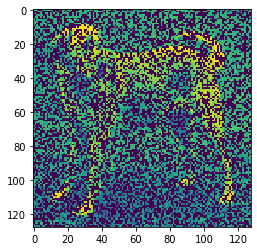# Binary weight backward function

I want to make an Autoencoder with binary weights at the first layer. You can see attached the network layout:

(Source)
I think I have succesfully implemented almost everything except the backward for binary layer. I am still a beginner in NN’s so I really need help to make this network better.
Here are my results right now:

But as you can see this is far from perfect. The problem is that I should also optimize the binary filters to get the best result. Here is a part of my code:

``````class BinaryFilterLayer(nn.Module):
def __init__(self,number_of_features):
super(BinaryFilterLayer, self).__init__()
self.number_of_features = number_of_features
# Generating the initial binary filters (only 0 and 1)
self.weight = nn.Parameter(torch.randint(high = 2,size=(1,self.number_of_features,128,128)).float())

def forward(self, input):
output = input * self.weight
return output
# here i need the backward but i dont really know how

class Model(nn.Module):
def __init__(self,encoding_dim):
super(Model, self).__init__()
self.encoding_dim = encoding_dim
# Encode
self.binary_filters = BinaryFilterLayer(self.encoding_dim)

# Decode
self.decoder = nn.Sequential(
nn.LayerNorm(self.encoding_dim),
nn.Linear(self.encoding_dim,128*128),
nn.LayerNorm(128*128),
nn.ReLU(),
Reshape(128,128),
nn.BatchNorm2d(64),
nn.ReLU(),
nn.BatchNorm2d(32),
nn.ReLU(),
nn.BatchNorm2d(1),
nn.Sigmoid()
)

def sumfunc(self,x):
x = torch.sum(x,dim=3)     # maybe there is an efficient way but this should work well also i guess
x = torch.sum(x,dim=2)
return x/(128*128)            # not sure if i need to normalize here but probably not

def forward(self, x):
x = self.binary_filters(x)  # multiply with binary layers
x = self.sumfunc(x)        # get the sum of each layer
x = self.decoder(x)        # decode
return x

``````

Can somebody help me to optimize these binary filters? Or is there a better way to make this network working better?

Here is a slight modification of the code

``````import torch
from torch import nn
from torchvision import transforms
import torchvision.models as models
import torch.nn.functional as F

class BinaryFilterLayer(nn.Module):
def __init__(self,number_of_features):
super(BinaryFilterLayer, self).__init__()
self.number_of_features = number_of_features
# Generating the initial binary filters (only 0 and 1)
self.weight = nn.Parameter(torch.randint(high = 2,size=(128,128)).float())
self.sigm=nn.Sigmoid()

def forward(self, input):
#print("size of input is {0} and size of weight is {1}".format(input.size(),self.weight.size()))
self.weight
output = input * torch.round(self.sigm(self.weight))
return output

class Flatten(nn.Module):
def forward(self, input):
return input.view(128,128)

class Model(nn.Module):
def __init__(self,encoding_dim):
super(Model, self).__init__()
self.encoding_dim = encoding_dim
# Encode
self.binary_filters = BinaryFilterLayer(self.encoding_dim)
# Decode
self.decoder = nn.Sequential(
#nn.LayerNorm(self.encoding_dim),
#nn.Linear(self.encoding_dim,128*128),
#nn.LayerNorm(128*128),
#nn.ReLU(),
#Flatten(),
nn.BatchNorm2d(64),
nn.ReLU(),
nn.BatchNorm2d(32),
nn.ReLU(),
nn.BatchNorm2d(1),
nn.Sigmoid()
)

def sumfunc(self,x):
x = torch.sum(x,dim=3)     # maybe there is an efficient way but this should work well also i guess
x = torch.sum(x,dim=2)
return x/(128*128)            # not sure if i need to normalize here but probably not

def forward(self, x):
x = self.binary_filters(x)  # multiply with binary layers
#print("Post binary filters, the size of x is {0}".format(x.size()))
#x = self.sumfunc(x)        # get the sum of each layer
x = self.decoder(x.view(1,1,128,128))        # decode
#print("After decoder the size of x is {0}".format(x.size()))
return x

model=Model(125)
criterion=torch.nn.MSELoss()
epochs=1000
from skimage import io
import cv2

for curEpoch in range(epochs):
loss.backward()
optimizer.step()
if(curEpoch %100==0):
print("Loss is {0}".format(loss.item()))
``````
``````import matplotlib.pyplot as plt
%matplotlib inline
plt.show()
``````Hope this helps

Thank you for your answer but maybe I was not clear with the question. You dont use the number_of_features variable which corresponds to the number of binary masks in the first layer. In the network, we want to generate M pieces of binary masks ((1,M,128,128)) and then the next layer is the sum of each images thus we will get an (1,M) size tensor. And what really confuses me is how to change the binary masks during the training because if they are only 0 and 1 than how can we define the derivative of these masks? At least for my code they wont change but this is the main point of this task to optimize the masks.
I hope now my question is more clearIn that case you can replace

`self.weight = nn.Parameter(torch.randint(high = 2,size=(128,128)).float())`

with

`self.weight = nn.Parameter(torch.randint(high = 2,size=(M,128,128)).float())`

This one is also not the right answer for my question. Right know I am trying to make a custom autograd function to optimize the binary masks but I have no idea how to calculate the forward and backward function in this case.